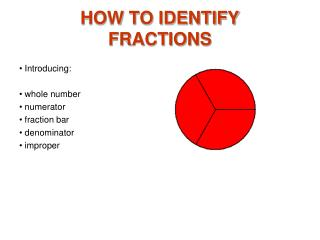DownloadDownload PresentationHOW TO IDENTIFY FRACTIONS

# HOW TO IDENTIFY FRACTIONS

Télécharger la présentation## HOW TO IDENTIFY FRACTIONS

- - - - - - - - - - - - - - - - - - - - - - - - - - - E N D - - - - - - - - - - - - - - - - - - - - - - - - - - -
##### Presentation Transcript

1. HOW TO IDENTIFY FRACTIONS Introducing: whole number numerator fraction bar denominator improper

2. The Whole Unit 1 A colored in circle is used to show one unit for a whole number 1.

3. The Whole Unit 2 Four circles show 4 units for a whole number 4.

4. The Whole Unit 3 This number line shows two units. The arrow and the red line shows that one unit is selected for a whole number 1.

5. The Whole Unit 4 The arrow and the red line shows that 3 units are selected for a whole number 3.

6. The Numerator and Denominator This unit has 5 equal parts.

7. The Numerator and Denominator Three of the parts are selected (colored).

8. The Numerator and Denominator The denominator 5 tells us that there are 5 equal parts in the unit. The numerator 3 tells us that 3 of the equal parts are selected (colored). This fraction can also be written as three-fifths.

9. The Numerator and Denominator There are 8 equal parts in this unit, giving a denominator of 8. Five of the parts are selected, giving a numerator of 5. This fraction can also be written as five-eighths.

10. The Numerator and Denominator The denominator 4 shows that the distance from 0 to 1 is divided into 4 equal parts. The numerator 1 shows that 1 of the parts is selected. This fraction can also be written as one-fourth.

11. The Numerator and Denominator The denominator 6 in the fraction 3/6 shows that the distance from 0 to 1 is divided into 6 equal parts. The numerator 3 shows that 3 of the 6 parts are selected. This fraction can also be written as three-sixths.

12. The Numerator and Denominator The numerator 4 shows that 4 of the 6 parts are selected. Notice the fraction increases in size as the numerator increases.

13. The Numerator and Denominator The denominator 3 tells us that the unit has 3 equal parts. One of the parts is selected for a numerator of 1. This fraction can also be written as one-third.

14. The Numerator and Denominator The denominator has been increased to 4. Notice the fraction has decreased in size.

15. The Numerator and Denominator The denominator has been increased to 5. As the denominator increases, the the fraction decreases in size.

16. The Numerator and Denominator The numerator increases to 2 and the fraction increases in size.

17. The Whole Number The numerator increases to 5 and the fraction increases to a complete unit. The fraction 5/5 is equal to whole number 1

18. The Mixed Number The numerator increases to 6. Now the fraction can be written as a mixed number. The mixed number 1 1/5 has a whole number part 1 and a fraction part 1/5.

19. The Mixed Number The picture shows that 6/5 can be written as 5/5 and 1/5 or 1 1/5. A fraction such as 6/5 where the numerator is the same or larger than the denominator is an improper fraction.

20. The Mixed Number The picture shows that 23/6 is made up of 6/6 + 6/6 + 6/6 + 6/6 + 5/6. Each 6/6 equals 1, so the fraction can be written as the mixed number 3 5/6.

21. The Mixed Number You can think of the numerator 23 as a dividend and the denominator 6 as a divisor. Dividing 23 by 6 will give a quotient of 3 with a remainder of 5. The quotient 3 is the whole number and the remainder 5 is the numerator in the mixed number 3 5/6 .